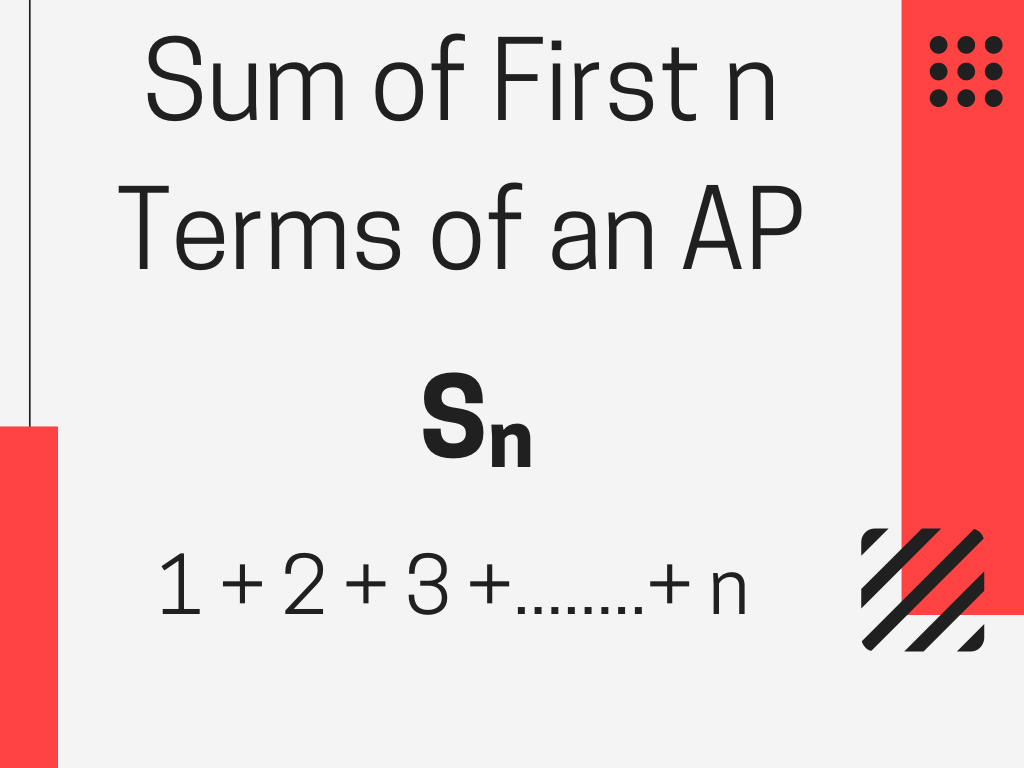## Sum of First n Terms of an Arithmetic Progression Class 10th

Introduction We know that there are n terms in an Arithmetic Progression (AP) and if we want the sum of the first n terms of an Arithmetic Progression then what should we do, we shall add all the terms of the AP but that will take more time to solve. Sometimes this method will not …